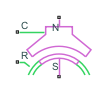# Rotating Air Gap

Air gap between stator tooth and rotating permanent magnet rotor

• Library:
• Simscape / Electrical / Electromechanical

•## Description

The Rotating Air Gap block models an air gap between a stator tooth and a rotating permanent magnet rotor. This block assumes that the rotor magnets are surface mounted and that the associated induced voltage is sinusoidal.

If the rotor angle is zero, specified by the Rotor angle variable in the Variables tab, then the rotor magnet perfectly aligns with the middle of the first stator tooth. The permanent magnet is then orientated to oppose the flux flow from port N to port S.

Use this block to create a magnetic representation of a permanent magnet synchronous motor (PMSM). For example, if you want to model a motor with nine stator poles, create nine copies of this block and set each of the Stator tooth reference index parameters to `1`, `2`, `3`, `4`, `5`, `6`, `7`, `8`, and `9`, respectively.

### Equations

This figure shows the equivalent circuit for the air gap and the adjacent permanent magnetwhere:

• ϕg is the magnetic flux that flows from the external magnetic circuit to port N.

• Rg is the air gap reluctance.

• mmf is the magnetomotive force across the rotating air gap component.

• Rm is the permanent magnet reluctance.

• ϕr is the magnetic flux generated by the rotor permanent magnets in the angle range subtended by the stator tooth.

This equation defines the relationship between ϕg, mmf, and ϕr:

`${\varphi }_{g}=\frac{mmf-{R}_{m}{\varphi }_{r}}{{R}_{m}+{R}_{g}}.$`

If the back EMF is sinusoidal, the flux density of the permanent magnet rotor is defined by this equation

`${B}_{r}={B}_{0}cos\left(N{\theta }_{s}-N{\theta }_{r}\right)$`

where:

• N is the Number of rotor pole pairs.

• θr is the rotor angle.

• θs is the stator angle.

• B0 is the Peak magnet flux density, in Tesla.

Then, to obtain the permanent magnet flux linkage, integrate over the stator angle subtended by the stator tooth

`${\varphi }_{r}\left({\theta }_{r}\right)=rl{\int }_{\frac{-{\theta }_{tooth}}{2}}^{\frac{{\theta }_{tooth}}{2}}\left[{B}_{0}\mathrm{cos}\left(N{\theta }_{s}-N{\theta }_{r}\right)\right]d{\theta }_{s}$`

where:

• r is the Rotor radius.

• l is the Tooth depth (in direction of shaft).

For an ideal PMSM, the θtooth must be equal to 2π/Ns, where Ns is the value of the Number of stator teeth parameter. Then the equation of the flux that flows through the equivalent circuit is obtained by solving the integral:

`${\varphi }_{r}\left({\theta }_{r}\right)=2{B}_{0}lr/Nsin\left(\frac{\pi N}{{N}_{s}}\right)\mathrm{cos}\left(N{\theta }_{r}\right).$`

To obtain the torque generated across the air gap, first calculate the total energy stored by the component:

`$E=\frac{1}{2}{\varphi }_{g}^{2}{R}_{g}+\frac{1}{2}{\left({\varphi }_{r}\left({\theta }_{r}\right)\right)}^{2}{R}_{m}.$`

Then, to obtain the torque, differentiate with respect to the rotor angle:

`$\tau =\frac{\partial E}{\partial {\theta }_{r}}=-2{B}_{0}{R}_{m}lrsin\left(\frac{\pi N}{{N}_{s}}\right)\mathrm{sin}\left(N{\theta }_{r}\right)\left({\varphi }_{g}+{\varphi }_{r}\left({\theta }_{r}\right)\right)/N.$`

Finally, calculate Rg and Rm in terms of geometry:

`$\begin{array}{l}{R}_{g}=\frac{g}{{\mu }_{0}{A}_{g}}\\ {R}_{m}=\frac{{l}_{m}}{{\mu }_{r}{\mu }_{0}{A}_{g}}\end{array}$`

where:

• μ0 is the permittivity of free space.

• μr is the relative permittivity of the permanent magnet.

• g is the Air gap.

• lm is the magnet length.

### Variables

To set the priority and initial target values for the block variables prior to simulation, use the Initial Targets section in the block dialog box or Property Inspector. For more information, see Set Priority and Initial Target for Block Variables.

Nominal values provide a way to specify the expected magnitude of a variable in a model. Using system scaling based on nominal values increases the simulation robustness. Nominal values can come from different sources, one of which is the Nominal Values section in the block dialog box or Property Inspector. For more information, see System Scaling by Nominal Values.

## Ports

### Conserving

expand all

Magnetic conserving port associated with the stator.

Magnetic conserving port associated with the rotor.

Mechanical rotational conserving port associated with the motor case.

Mechanical rotational conserving port associated with the motor rotor.

## Parameters

expand all

Number of the pole pairs of the rotor. This parameter must be equal to or greater than `1`.

Number of teeth of the stator. This parameter must be equal to or greater than `3`.

Reference index of the stator tooth of the motor. This parameter must be between `1` and the value of the Number of stator teeth parameter.

For example, if you want to model a motor with nine stator poles, create nine copies of this block and set the Stator tooth reference index parameter for each of the Rotating Air Gap blocks to `1`, `2`, `3`, `4`, `5`, `6`, `7`, `8`, and `9`, respectively.

Peak flux density associated with the permanent magnet rotor. The flux density is sinusoidal with the rotor angle.

Length of the magnet in the radial machine direction or, equivalently, in the direction of the magnetic flux. This parameter must be less than the value of the Rotor radius parameter.

Relative permeability of the permanent magnets. Typically, you should set this value a little greater than `1` to reflect that the magnetic dipoles are already aligned in a permanent magnet.

Length of the air gap in the radial direction.